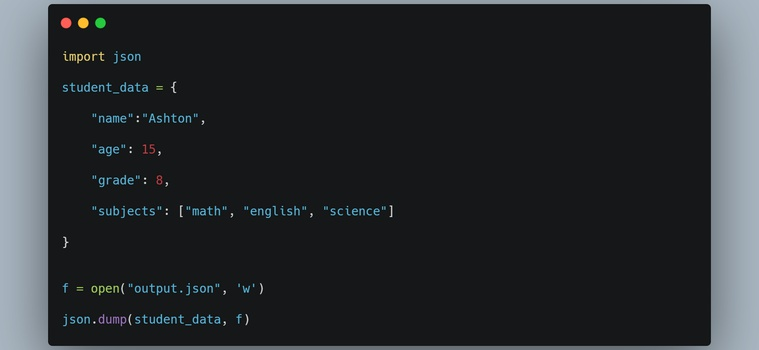# How to work with JSON Data in Python

March 17, 2021, 10:07 p.m.In this article, we will learn how to work with JSON data in Python. JSON stands for JavaScript Object Notation, and it is a popular text-based data format. Even though JSON was derived from JS, it has been language-independent for quite some time now. Therefore, it can be used by any programming language. It is used to store and exchange data. For example, when the data is fetched from an API or used in some document database, it is mostly in JSON format. Let’s take an example.

``````{

"name":"Ashton",

"age":15,

"subjects":["math", "english", "science"],

}``````

As you can see, the above data is very readable and looks a lot like a Python dictionary. A data item consists of a key/ value pair containing a colon (:) in between them. Moreover, two data items are separated by a comma (,).

With the intro out of the way, let’s get started and see how to handle JSON data in Python.

Python has native support for JSON and provides a built-in module JSON for that purpose. Add the following line at the top of your code to start working with JSON.

``import json``

First, let’s look at how to perform serialization, i.e., encoding JSON data.

Encoding JSON data (Python to JSON)

Writing JSON to a file

The JSON module provides the dump() method to write JSON data to a file. It takes two required arguments, a Python object to be encoded and a file-like instance. Consider the following example.

``````import json

student_data = {

"name":"Ashton",

"age": 15,

"subjects": ["math", "english", "science"]

}

f = open("output.json", 'w')

json.dump(student_data, f)``````

Output

As you can see in the above example, we have a Python dictionary containing student’s information such as name, age, grade, and subjects. We open the output.json file in the write mode and dump the student_data object to a JSON file.

Consider the following table that shows the conversion of Python objects to JSON.

 Python JSON dict object int float number str string list tuple array True true False false None null

You can also write JSON data in a prettier format using some arguments. For example, the indent argument can take a non-negative integer or a string to specify the indentation value. If you pass 0, a negative value, or an empty string, dump() will only insert newlines. Moreover, setting None (the default value) results in the most compact representation. You can also sort the data by keys by passing True to the sort_keys argument. By default, it is False. Let’s take an example.

``````import json

student_data = {

"name":"Ashton",

"age": 15,

"subjects": ["math", "english", "science"]

}

f = open("output.json", 'w')

json.dump(student_data, f, indent=2, sort_keys=True)``````

Output

{

"age": 15,

"name": "Ashton",

"subjects": " [

"math",

"english",

"science",

]

}

As you can see in the above output, it is cleaner and more human-readable.

Convert a Python Object to a JSON String

To convert a serialized object (such as a dictionary) to a JSON string, you can use the dumps() method. It is similar to dump(), except it does not require a file object, and it returns a string containing the information in JSON format. Let’s see.

``````import json

student_data = {

"name":"Ashton",

"age": 15,

"subjects": ["math", "english", "science"]

}

json_string = json.dumps(student_data, indent=2, sort_keys=True)

print(json_string)

print(type(json_string))``````

Output

{

"age": 15,

"name": "Ashton",

"subjects": [

"math",

"english",

"science"

]

}

<class 'str'>

Let’s now go ahead and see how to perform deserialization, i.e., decoding JSON data.

Decoding JSON data (JSON to Python)

Parse a JSON File

The JSON module provides the load() method to load JSON from a file to a Python object. It takes a file-like object as its required argument. Consider the following example.

``````import json

f = open('sample.json', 'r')

print(data)

print(type(data))``````

Output

{'name': 'Ashton', 'age': 15, 'grade': 8, 'address': None, subjects': ['math', 'english', 'science']}

<class 'dict'>

The conversion rules from JSON to Python are given in the table below.

 JSON Python object dict integer number int real number float string str array list true True false False null None

Parse a JSON String to an Object

Similarly, you can use the loads() method to convert a JSON string to a Python object. It takes the string containing a JSON document as its required argument. Let’s see.

``````import json

json_str = '''{

"name":"Ashton",

"age":15,

"subjects":["math", "english", "science"]

}'''

print(data)

print(type(data))``````

Output

['math', 'english', 'science']}

<class 'dict'>

Here, the json_str variable contains a JSON document in a multiline string. It gets parsed into a dictionary using the loads() method.

One more thing to note here is that if the Python object is serialized and then deserialized, it may necessarily not be equal to the original one. Consider the following example.

``````import json

student_data = {

"name":"Ashton",

"age": 15,

"subjects": ("math", "english", "science")

}

json_string = json.dumps(student_data, indent=2, sort_keys=True)

print(student_data==parsed_data)

``````

Output

False

In the above example, student_data contains key subjects whose value is of the type tuple. When student_data gets serialized to a JSON string, subjects’ value gets converted to an array according to the conversion rule. When we perform decoding, it gets transformed to a list and not to a tuple. Therefore, the original data and the decoded one are not equal.